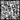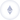Search by

## 如何在证明中使用 KZG 承诺

本文介绍了如何在 zk 证明中有效地使用 KZG 承诺，提高证明验证的效率。

DF

翻译 | Renaissance

校对 | doublespending，Franci

感谢 ECN 翻译志愿者 Renaissance 和 doublespending 对本文的贡献！

在 Discord 私信 ECN “EthereumCN#9778”，加入 ECN 内容生成者联盟！

EIP-4844 将一个新的对象引入到以太坊：使用 KZG 承诺对额外的 calldata 进行承诺

$C = [f(s)]_1$

其中，$f$ 是一个函数，用来评估在一些固定点 (4096 阶单位根) 上插值数据，$s$ 是 KZG 受信任初始化秘密，$[x]_1=xg_1$$G_1$ 元素的简写。

函数 $f$ 被定义为插值多项式

$f(w^i)=d_i$

$ω^{4096} = 1$，w 是 4096 阶单位根，$d_i$定义数据点。（或者，应用可以忘记$d_i$，简单地将多项式本身视为输入）。

以太坊数据交易仅能获取 KZG 承诺 C 用作输入，且无法直接访问数据。提供的唯一访问方式是验证评估的预编译函数，例如给定 $C$$x$ 和 $y$, 验证 $f(x)=y$

当前，Optimistic rollup倾向于使用多轮挑战，因此任何欺诈声明最终总能通过归纳到 f(x) 上的一个点上的分歧来解决。然而，ZKRollups 如何做到同样的事情呢？

对本来就基于 KZG 承诺证明的方案很容易，例如使用 BLS12_381 的 PLONK。然而，许多应用会选择不同的证明方案，更重要的是，会选择其他具有不同群阶的椭圆曲线，并因而产生其他域。。这在证明中计算 KZG 承诺将非常昂贵（4096 BLS12_381 操作）。我们怎样才能降低这个成本？

## 取巧方案

最初的取巧方案是众所周知的；在同样的域上，给定两个多项式承诺$C1$ and $C2$ （但不是同一个方案，例如它们可以是 KZG 和 FRI，或者都是 KZG，但具有不同的受信任初始化方案），存在有效的方式可以证明2个多项式等价，例如对相同的$f(x)$进行承诺:

1. 设 $x=hash(C_1,C_2)$
2. 计算 $y=f(x)$
3. 产生证明 $π_1$, 可以证明与多项式$C_1$对应的 $y=f(x)$
4. 产生证明$π_2$, 可以证明与多项式$C_2$对应的 $y=f(x)$

证明者发送 $C_1$$C_2$$y$$π_1$$π_2$，如果 $y=f(hash(C_1,C_2))$ 并且证明$π_1$ 和 $π_2$ 被验证通过，验证者则接受。

如果域足够大（256 位的域可以得到 128 位级别的安全型），则该方案的正确性来自 Schwarz-Zippel 理论。

## 非对齐域上的 ZKP

前面看到的取巧方案，只有$C_1$ 和 $C_2$多项式在同样的域上才能有效工作。但是大多数证明系统在不同域上工作。所以在这种形式下它没有那么有用。

然而，也并非全然没用。有两种方法可以通过将 kzg 承诺数据引入到一个证明之中来设计出高效可行的机制。

### 方法一：使用默克尔树

一颗叶子为 $d0$,…,$d_{4095}$ 的 Merkle 树实际上就是一个多项式承诺。为了评估一个点，证明者简单把点$d0$,…,$d_{4095}$ 给到验证者。验证者可以基于这些点重构$f(x)$ 并检查是否$f(x)=y$

这样做的缺点是产生的证明庞大，但在我们的场景中还好：这个证明只是证明的见证，我们希望电路能够访问数据 $d_0$,…,$d_{4095}$ 。剩下的就是评估这些点的成本。使用barycentric formula，可以使用 4096 次乘法和 4096 次除法来完成。

总成本 将数据引入电路的全部成本包括 (1) Merkle 树计算，需要 4095 次哈希和 (2) 8192 个非对齐域操作（乘法和除法）。

自从引入像 Plookup 这样的方案以来，非对齐的域操作成本已经下降了很多。

### 方法二：使用“Fiat 输入”

“fiat 输入”是为了如下构造尝试提出的名字，：让 e0,…,e_{k-1} 是域元素，通过一种 “合适的” 承诺方案对这些元素进行承诺。“合适的”意味着它在某种形式上与证明方案兼容。给定承诺设为 $E$

之所以对电路来说 $e_0$,…,$e_{k−1}$ 是“fiat输入”，意思是域元素 $e_0$,…,$e_{k−1}$ 具有和输入线一样的可用性，以及其对应的一条包含 E 的线（也可能是为了用一个域元素表示而被截断的承诺）。

下面我将证明使用 KZG 承诺向 PLONK 添加 “Fiat输入” 很容易，只需让验证者增加少量工作即可。特别是，需要的工作量是恒定的，并不与$k$成比例关系。相信这种构造可以推广到几乎任何证明者方案，但也确实需要对方案进行一些修改。

首先看下如果我们有“Fiat input”能做什么：让我们添加数据$d_i$作为 fiat 输入。如果证明域比BLS（Barreto Lynn Scott）域更大，可以设定$e_i=d_i$并留下一些前导零。如果是更小，就需要用几个$e$域元素来编码一个$d$ BLS 元素。

现在就可以在$C_1$ 和 $C_2$上，设定$C_2=E$ 并执行多项式承诺等效性证明了。

总成本 在电路内部，我们现在只需评估多项式，即 8192 个非对齐域操作。不需要哈希（除了单独一个用于计算随机的点$x$需要外）。

如果电路中的执行哈希很昂贵，则此方法有很大的优势。如果出于安全原因不应使用算术哈希函数，则大多数情况下会出现这种情况；如果可以使用算术哈希函数，则哈希成本可能与评估成本相似，并且这种技术的优点不值得把方案复杂化。

## 在 PLONK 中构建 Fiat 输入

为了展示可以构造具有上述属性的Fiat输入，我接下来将分析如何修改 PLONK 协议以添加Fiat输入。这最好被描述为对 PLONK 协议（特别是基于 KZG 的 PLONK 协议）的一个小修改，因此请对着 PLONK 的论文阅读本节。

我们想要添加 Fiat 输入 $e=e_0$,…,$e_{k−1}$ 到电路。意味着我们想把输入 $e=e_0$,…,$e_{k−1}$  以及我们将对 E 应用 map_to_field(E)。

请注意，PLONK 保留了电路中的 ℓ 公共输入。我们将占用 (abuse) 前 k+1 公共输入用作 fiat 输入。其工作流程如下：

对于证明者，我们添加以下步骤：

1. 通过对函数$FI(X)=∑^{k−1}_{i=0}e_iL_i(X)$$E=[FI(s)]_1$应用KZG 承诺，证明者对 fiat 输入进行承诺。
2. 作为证明的一部分，证明者添加$E$以及一个KZG证明$π$，表明域上除了$i=0,…,k−1$之外满足$FI(X)$为0。

对于验证者，我们添加以下步骤：

1. 验证证明 $π$，即在域中除$i=0,…,−1$满足$FI(X)$ 为0。
2. 当计算公共输入时，需要添加已经“被占用”的Fiat 输入。出于这个目的，公共输入多项式就变成了 $PI(X)=−FI(X)−map\_to\_field(E)L_k(X)−∑^{ℓ−1}_{i=k+1}x_iL_i(X)$. 实际上，验证者并不知道多项式，但是可以计算承诺 $[FI(s)]_1=−E−[−map\_to\_field(E)L_k(S)−∑^{ℓ−1}_{i=k+1}x_iL_i(s)]_1$ ，这已经足够完成对证明的验证。

这足以将Fiat输入添加到 PLONK；事实上，与这个想法相似的，任何使用加法同态承诺的方案都应该是有效的。如果没有加法同态承诺，事情会变得有点困难，但至少只要承诺是有效的，仍然有办法获得Fiat输入。

ECN 的翻译工作旨在为以太坊中文社区传递优质资讯和学习资源，文章版权归原作者所有，转载须注明原文出处以及ethereum.cn，若需长期转载，请联系eth@ecn.co 进行授权。

Ethereum Community Network
以太坊社区网络Ethereum Community Network
以太坊社区网络